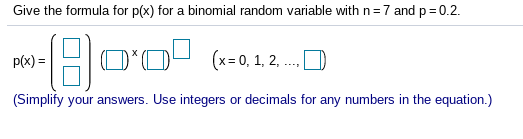# Give the formula for p(x) for a binomial random variable with n 7 and p0.2O(-.1.2.(x=0, 1, 2,p(x)(Simplify your answers. Use integers or decimals for any numbers in the equation.)

Question
12 views

I have the binomial table I just have no idea what they're asking forhelp_outlineImage TranscriptioncloseGive the formula for p(x) for a binomial random variable with n 7 and p0.2 O(-.1.2. (x=0, 1, 2, p(x) (Simplify your answers. Use integers or decimals for any numbers in the equation.) fullscreen
check_circle

star
star
star
star
star
1 Rating
Step 1

The provided information is:

n=7

p=0.2

Consider X is a binomial random variable. n is number tri...

### Want to see the full answer?

See Solution

#### Want to see this answer and more?

Solutions are written by subject experts who are available 24/7. Questions are typically answered within 1 hour.*

See Solution
*Response times may vary by subject and question.
Tagged in

### Statistics